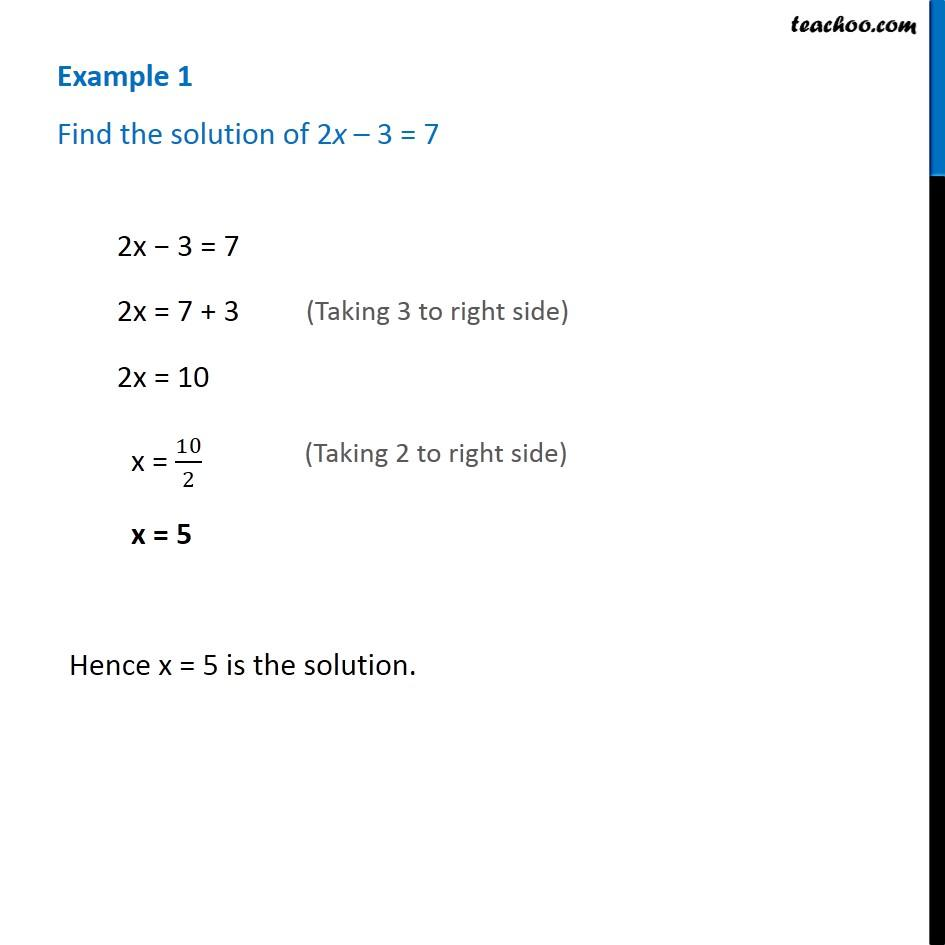Examples

Chapter 2 Class 8 Linear Equations in One Variable
Serial order wiseLearn in your speed, with individual attention - Teachoo Maths 1-on-1 Class

### Transcript

Question 1 - Chapter 2 Class 8 Linear Equations in one variable - NCERT Book Find the solution of 2x – 3 = 7 2x − 3 = 7 2x = 7 + 3 (Taking 3 to right side) 2x = 10 x = 10/2 (Taking 2 to right side) x = 5 Hence x = 5 is the solution.